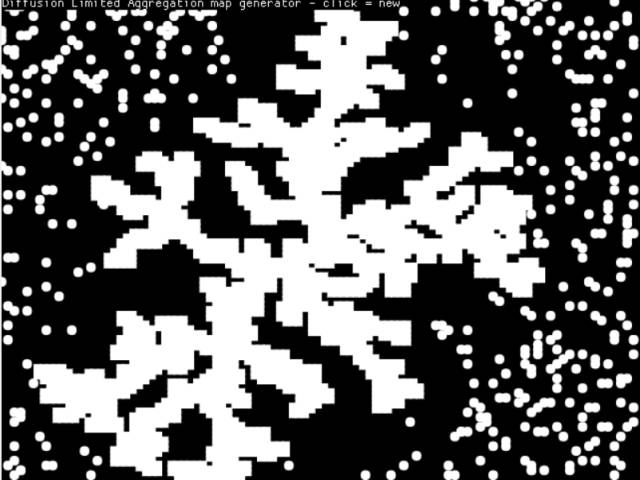-=+=- -=+=- -=+=- -=+=- -=+=- -=+=- -=+=- -=+=- -=+=- -=+=- -=+=- -=+=- -=+=- -=+=- -=+=- -=+=- -=+=- -=+=- -=+=- -=+=- -=+=- -=+=- -=+=- -=+=- -=+=- -=+=- -=+=- -=+=- -=+=- -=+=- (c) WidthPadding Industries 1987 0|362|0 -=+=- -=+=- -=+=- -=+=- -=+=- -=+=- -=+=- -=+=- -=+=- -=+=- -=+=- -=+=- -=+=- -=+=- -=+=- -=+=- -=+=- -=+=- -=+=- -=+=- -=+=- -=+=- -=+=- -=+=- -=+=- -=+=- -=+=- -=+=- -=+=- -=+=-
SoCoder -> Snippet Home -> Generators

PakzCreated : 01 August 2017
Edited : 01 August 2017
Language : Monkey-X

### Diffusion Limited Aggregation Maps

Coding challenge from the coding train

Flash applet of the dla
Screenshots## Latest Update

What this code does is create images by putting random walkers on the screen and one solid point. When a random walker touches a solid point he turns into a solid point, and so on. This creates some kind of natural image we see in coral reefs ect. It is some kind natural thing.